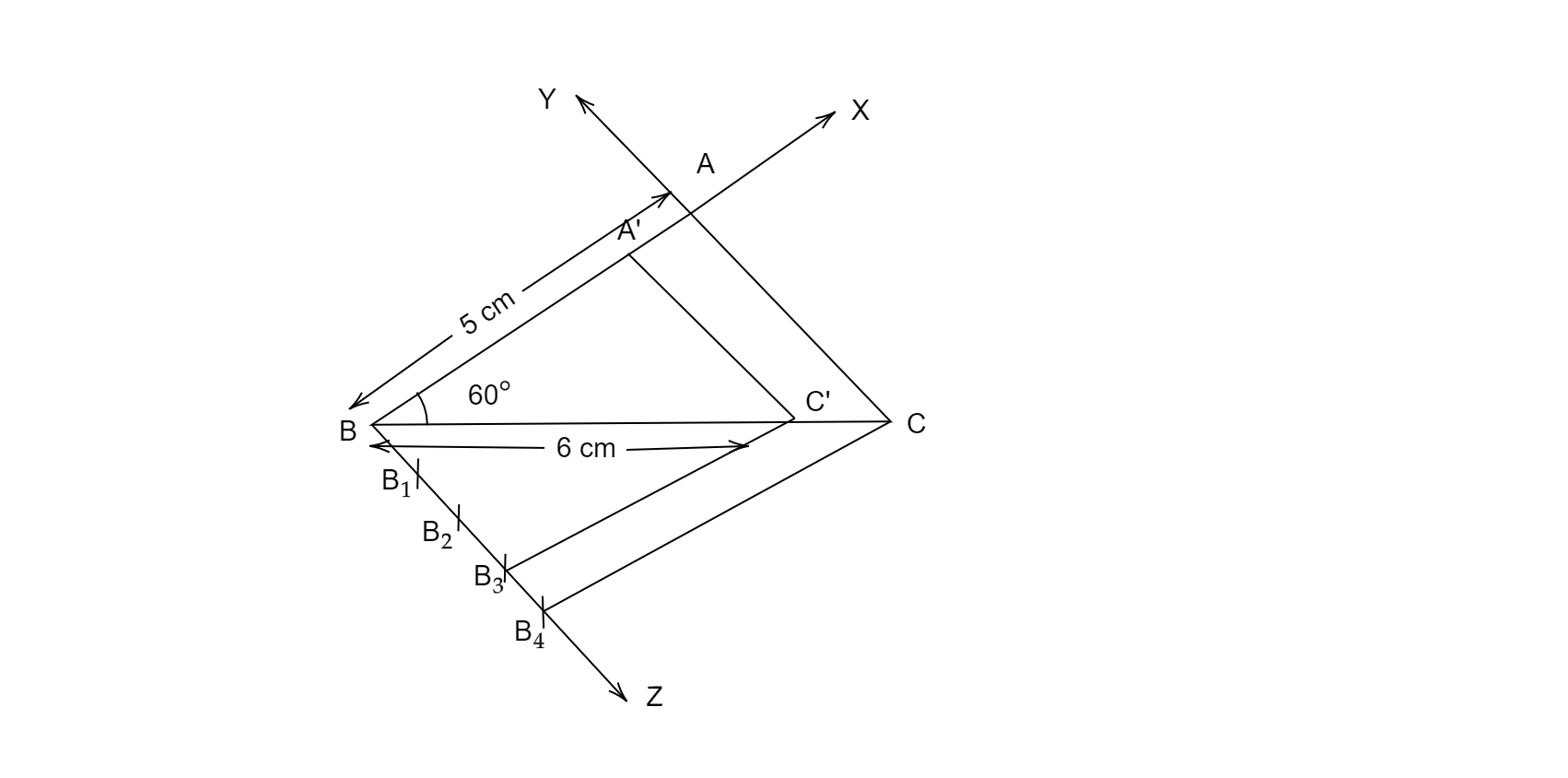# Draw a triangle ABC with $BC = 6\ cm, AB = 5\ cm$ and $\vartriangle ABC=60^{o}$. Then construct a triangle whose sides are $\frac{3}{4}$ of the corresponding sides of the ABC.

Given: $BC = 6\ cm, AB = 5\ cm$ and  $\angle ABC=60^{o}$.

To do: To draw $\vartriangle ABC$ and then to construct  a triangle whose sides are $\frac{3}{4}$ of the corresponding sides of the ABC.

Solution:

STEPS OF CONSTRUCTION:

$( i)$ Draw a line segment $BC = 6\ cm$, draw a ray $BX$ making $60^{o}$ with $BC$.

$( ii)$ Draw an arc with radius $5\ cm$ from $B$ so that it cuts $BX$ at $A$.

$( iii)$ Now join AC to form $\vartriangle ABC$.$( iv)$ Draw a ray by making an acute angle with NC opposite to vertex A.

$( v)$ Locate 4 points  on by such that $BB_{1}=B_{1}B_{2}=B_{2}B_{3}=B_{3}B_{4}$

$(vi)$ Join $B_{4}$  to $C$.

And now draw a line from $B_{3}$ parallel to $B_{4}C$. so that it cuts $BC$ at $C'$.

$( vii)$ From C draw a line parallel to AC and cuts AB at A' .

$( viii)\vartriangle A' BC '$  is the required triangle.

Updated on: 10-Oct-2022

27 Views